# 3D Plots in MATLAB

In MATLAB, we can plot different types of modules like 2d plotting and 3d plotting. In this article, we will see what are the various types of 3D plotting.

• Mesh Plot: A mesh plot is a 3d surface that creates different types of meshes for different types of expression. To create mesh we have to give the values x and y for z, (z= f(x, y)). For plotting the mesh plot it has mesh() which will generate the 3d surface. It has solid edge color but no face color.

Syntax:

`mesh(Z)`

Example:

## Matlab

 `% give the input of x and y. ` `[x,y]= meshgrid(0:0.1:5); ` ` `  `% give the expression for x  ` `% and y for the output in z ` `z= sin(x).*cos(y); ` ` `  `% mesh() is used for 3D plotting ` `mesh(z);`

Output: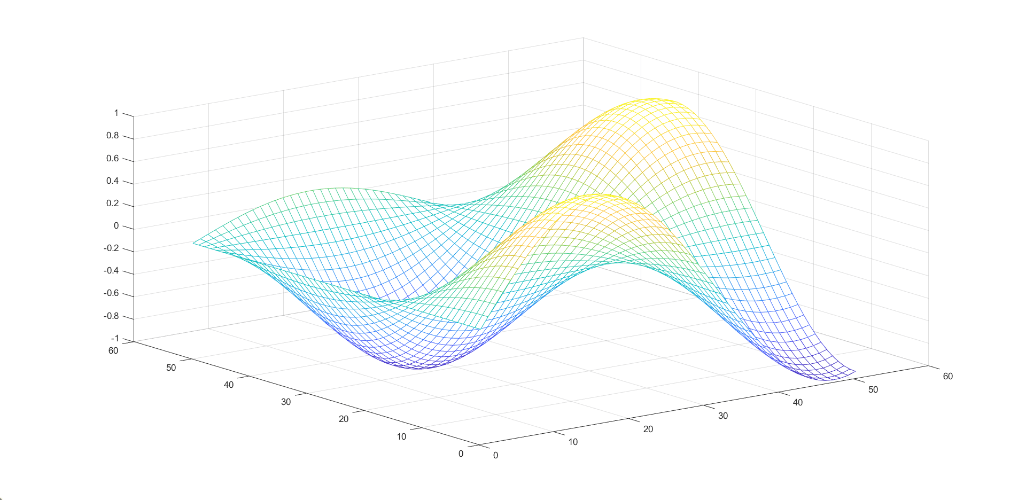• Surface plot: A surface plot is a 3d surface that creates different types of surfaces for different expressions. To create a surface we have to give the values x and y for z, (z= f(x, y)). For plotting the surface plot it has surf() which will generate the 3d surface. It has solid edge color and solid face color

Syntax:

`surf(Z)`

Example:

## Matlab

 `% give the input for x and y ` `[x,y]= meshgrid(0:0.1:5); ` ` `  `% give the expression for  ` `% x and y for the value of z. ` `z= sin(x).*cos(y); ` ` `  `% use surf() for the plotting  ` `surf(z)`

Output: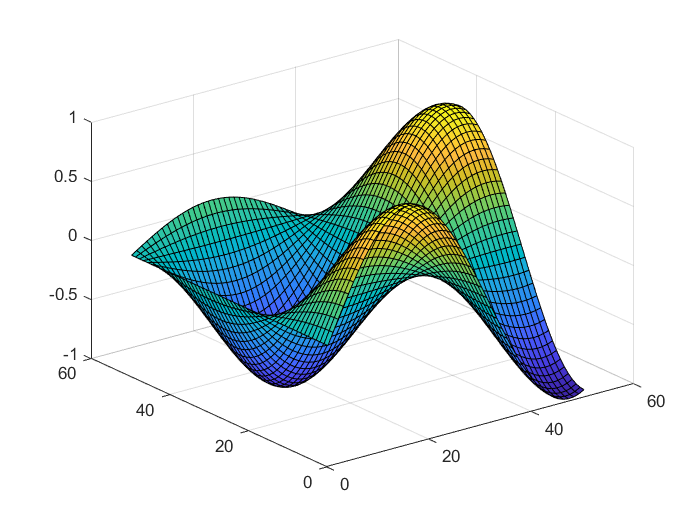• Surface plot(with shading): A surface plot that creates a three-dimensional surface plot that has solid edge colors and solid face colors and also has shading. In surface with shading, we have to give the values x and y for z, (z= f(x, y)).  For plotting the surface plot it has surf(z) is used for 3d plotting.

Syntax:

`surfl(z)`

There are three types of shading available:

Example:

## Matlab

 `% give the input for x and y ` `[x,y]= meshgrid(0:0.1:5); ` ` `  `% give the expression for x and y  ` `% for the value of z ` `z= sin(x).*cos(y); ` ` `  `% use surfl() for the plotting  ` `% shading faceted is by default ` `surfl(z) ` `shading faceted ` `title(``'Faceted Shading'``) ` ` `  `% use shading flat for each mesh ` `% line segment and face has a  ` `% constant color  ` `surfl(z) ` `shading flat ` `title(``'Flat Shading'``) ` ` `  `% use shading flat for varies the  ` `% color in each line segment and  ` `% face by interpolating ` `surfl(z) ` `shading interp ` `title(``'Interpolated Shading'``) `

Output: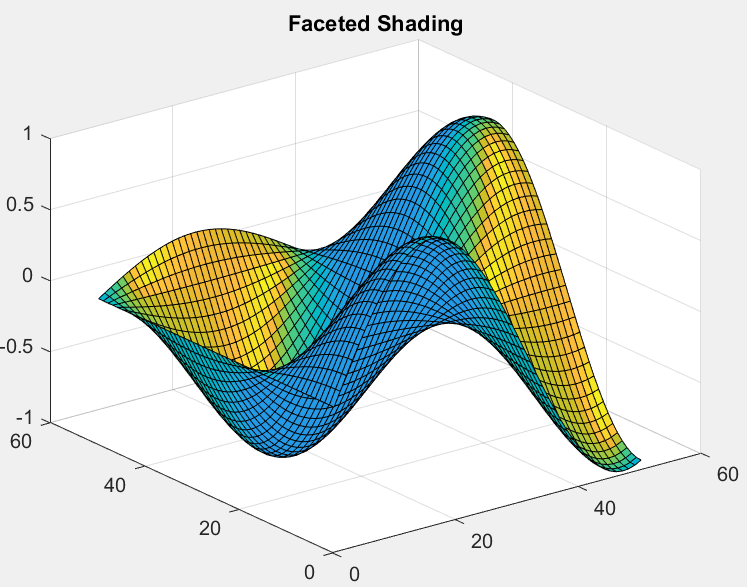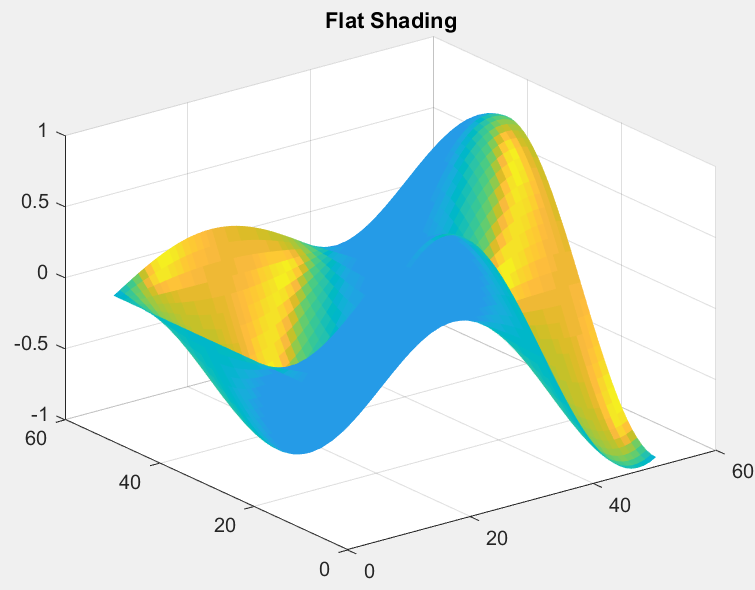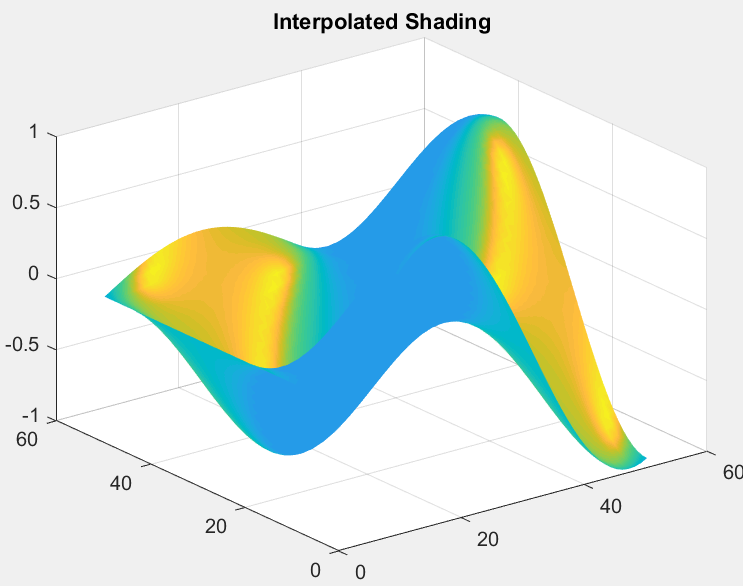• Contour plot: A contour plot is also called a line plot. To plot contour it has x, y variables which are used to give the values for z, (z=f(x, y)). The x and y variables are usually in a grid called meshgrid.

Syntax:

`contour(Z)`

Example:

## Matlab

 `% enter the inputs of x and y ` `[x,y]= meshgrid(0:0.1:5); ` ` `  `% enter the expression using ` `% x and y  ` `z= sin(x).*cos(y); ` ` `  `% use contour() for plotting  ` `contour3(z,50)`

Output: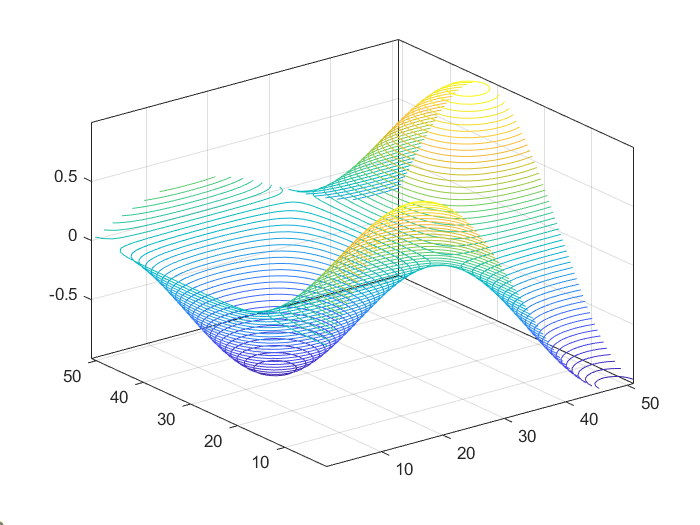• Quiver plot: A quiver plot or vector plot is a type of plotting that gives directional components of u, v, w using the cartesian components x, y, and z. For plotting of quiver plot use quiver3().

Syntax:

`quiver3(X, Y, Z, U, V, W)`

Example:

## Matlab

 `% give the input value for x, ` `% y and z ` `[x,y,z]= meshgrid(0:0.1:5); ` ` `  `% using x, y and z give the  ` `% values for u,v and w  ` `u= sin(x).*cos(y); ` `v= sin(x).*cos(y); ` `w= sin(x).*cos(y); ` ` `  `%use quiver3() for 3d plotting ` `quiver3(x,y,z,u,v,w); `

Output: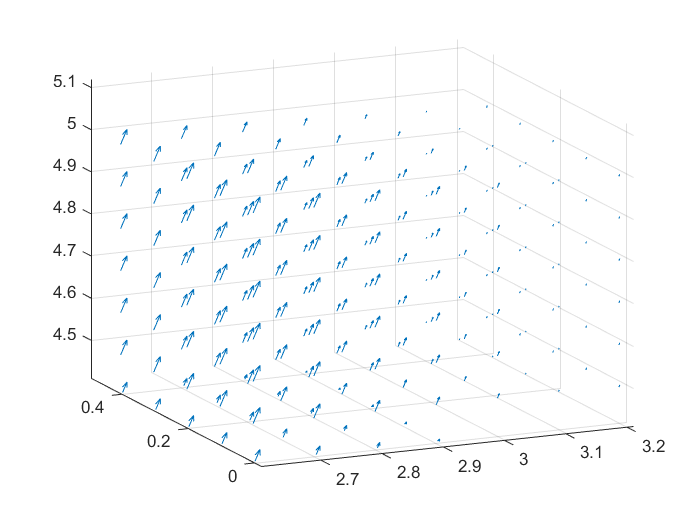Whether you're preparing for your first job interview or aiming to upskill in this ever-evolving tech landscape, GeeksforGeeks Courses are your key to success. We provide top-quality content at affordable prices, all geared towards accelerating your growth in a time-bound manner. Join the millions we've already empowered, and we're here to do the same for you. Don't miss out - check it out now!

Previous
Next